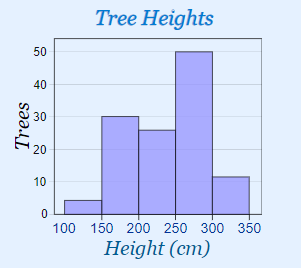What Are Histograms? Among the different graphs provided to us by statistics, the histogram is the most widely used one. This special form of bar chart enables us to represent data comprehensively in discrete categories. In other words, the histogram does not have any gap between the columns to represent different categories. It is similar to a bar chart, but histogram groups numbers in the form of ranges. The height of each bar shows how many fall into each range. Let us consider an example to find the height of orange trees in the orchard. The heights lie between 100 cm to 350 cm. We will decide to put the results into a group of 50 cm. Now, let us look at the graph we made.In the graph, you can see that there are 30 trees from 150 cm to just below 200 cm tall.

• ### Basic Lesson

Using a snap shot of random data, students learn to read histograms. The discounts offered by super market are shown in the table. Represent the data in the histogram. 1. Set up the bottom axis (x axis- Amount). Look at total range of values and check the lowest value. Plot the range of values on axis. 2. Set up side axis (Y axisDiscount). Group up the values on the similar range of X axis (Amount). 3. Construct data bars centered over X axis.

• ### Intermediate Lesson

Students begin to read large histograms.

• ### Independent Practice 1

Students interpret 20 histograms.

• ### Independent Practice 2

Students interpret another 20 histograms.

• ### Homework Worksheet

Offers 12 questions for home and provides an example.

• ### Skill Quiz

Students use a series of histograms to answer 10 quiz questions.

• ### Homework and Quiz Answer Key

Answers for the homework and quiz.